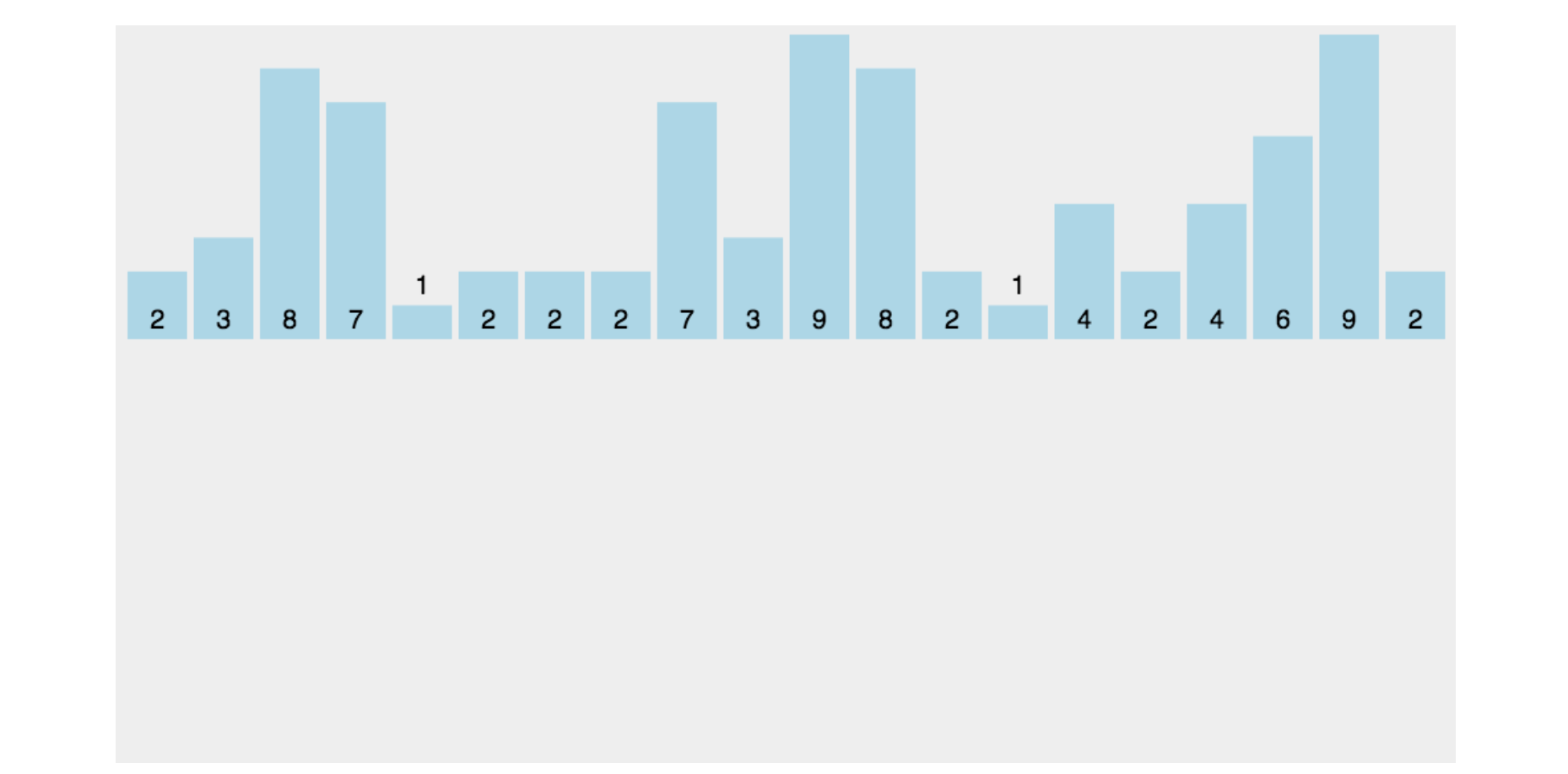# 什么是计数排序？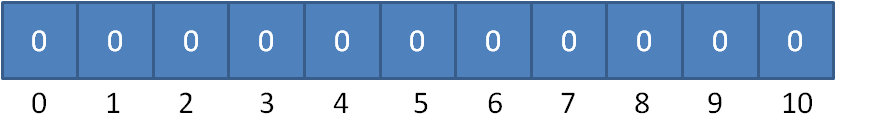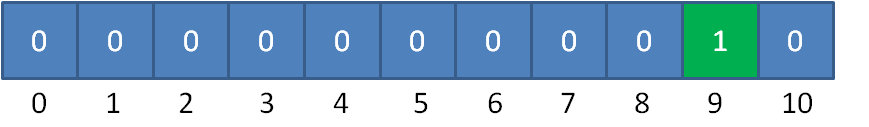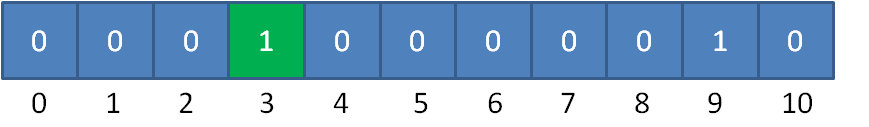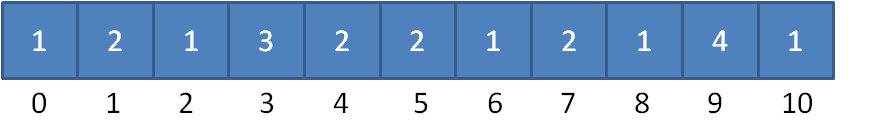0，1，1，2，3，3，3，4，4，5，5，6，7，7，8，9，9，9，9，10

Java 代码如下（代码会做详细的注释）

   public static int[] countSort(int[] arr) {
if(arr == null || arr.length < 2) return arr;

int n = arr.length;
int max = arr;
// 寻找数组的最大值，该值用来创建临时数组用的
for (int i = 1; i < n; i++) {
if(max < arr[i])
max = arr[i];
}
//创建大小为max + 1的临时数组
int[] temp = new int[max + 1];
//统计元素i出现的次数
for (int i = 0; i < n; i++) {
temp[arr[i]]++;
}
int k = 0;
//把临时数组统计好的数据汇总到原数组
for (int i = 0; i <= max; i++) {
// temp[i] 的值表示元素 i 出现的次数
for (int j = temp[i]; j > 0; j--) {
arr[k++] = i;
}
}
return arr;
}


    public static int[] sort(int[] arr) {
if(arr == null || arr.length < 2) return arr;

int n = arr.length;
int min = arr;
int max = arr;
// 寻找数组的最大值与最小值
for (int i = 1; i < n; i++) {
if(max < arr[i])
max = arr[i];
if(min > arr[i])
min = arr[i];
}
int d = max - min + 1;
//创建大小为max的临时数组
int[] temp = new int[d];
//统计元素i出现的次数
for (int i = 0; i < n; i++) {
temp[arr[i] - min]++;
}
int k = 0;
//把临时数组统计好的数据汇总到原数组
for (int i = 0; i < d; i++) {
// temp[i] 的值表示元素 i 出现的次数
for (int j = temp[i]; j > 0; j--) {
arr[k++] = i + min;
}
}
return arr;
}